### Explanation of the 4x4 (pan)magic square

What kind of 4x4 magic squares are there?

Excluding rotation and/or mirroring there are 880 different pure magic 4x4 squares. On the website of Harvey Heinz the 4x4 magic squares are divided into 12 groups. Within a group all magic squares have the same structure.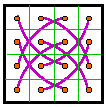Group 1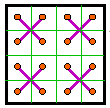Group 2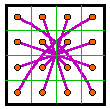Group 3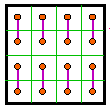Group 4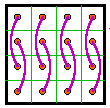Group 5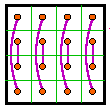Group 6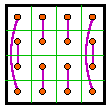Group 7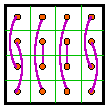Group 8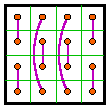Group 9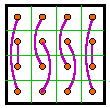Group 10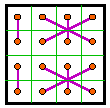Group 11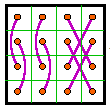Group 12

De lines are connecting the numbers 1-16, 2-15, 3-14, 4-13, 5-12, 6-11, 7-10 and 8-9

(1+16 = 2+15 = 3+14 = 4+13 = 5+12 = 6+11 =7+10 = 8+9 = 17, that is half of the magic sum of 34).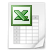4x4, analysis.xls

What is a 4x4 magic square?

The 4x4 magic square is square, because it has as many rows (from left to right = horizontal) as columns (from top to bottom = vertical).

The 4x4 magic square consists of 4 rows which multiplied by 4 columns is 16 cells.

The 4x4 magic square must contain 16 different numbers. A pure magic 4x4 square contains the numbers 1, 2, 3, 4, 5, 6, 7, 8, 9, 10, 11, 12, 13, 14, 15 and 16.

The magic square is magic, because the sum of the numbers of each row, each column and both diagonals always give the same result. The sum can be calculated as follows, the (even) size of the square divided by 2 multiplied by the lowest plus the highest number: 4 / 2 x (1 + 16) = 34.

What is a 4x4 panmagic square?

A 4x4 panmagic square has the above mentioned row-, column- and diagonal features (= minimum features) plus extra magic features.

The extra magic features of the panmagic 4x4 square are:

• Each 2x2 sub-square has the same (magic) sum (= 34);
• Each 4x4 sub-square on a carpet of 2x2 (see below) is panmagic (in the carpet you can find 16 different 4x4 panmagic squares).

Each 4x4 subsquare on the 2x2 carpet is panmagic, because of the parallel running diagonals on the carpet. The sum of the numbers of four sequencing cells on a diagonal is always 34 (= the magic sum).

(pan)diagonals from left to right                 (pan)diagonals from right to left

Notify that in a 4x4 sub-square not only the sum of the four numbers of the ordinary diagonals but also the sum of the four numbers of the (six) broken diagonals (= pandiagonals) is 34; see below.

 1 8 13 12 1 8 13 12 15 10 3 6 15 10 3 6 4 5 16 9 4 5 16 9 14 11 2 7 14 11 2 7

What is the secret behind the panmagic 4x4 square

How to get each time the same (magic) sum? Add the the lowest number to the highest number, the second lowest number to the second highest number, the third lowest number to the third highest number, …. If you split up the numbers of the 4x4 square in 1 up to 8 and 9 up to 16, than you get:

1+8 = 2+7 = 3+6 = 4+5 = 9   and   9+16 = 10+15 = 11+14 = 12+13 = 25

See the panmagic 4x4 square below.

 1 8 13 12 15 10 3 6 4 5 16 9 14 11 2 7

Each row consists of 9 + 25 = the magic sum of 34.

 1 8 13 12 15 10 3 6 4 5 16 9 14 11 2 7

Each column consists of 16 + 18 = 34

N.B.: The square is panmagic because of the following structure (= the structure of all most perfect magic squares that are multiples of 4; 4x4, 8x8, 12x12, …):

 1 8 13 12 15 10 3 6 4 5 16 9 14 11 2 7

The sum of two numbers of the same colour is each time (the highest number of the magic square + 1, in this case 16+1=) 17, that is half of the magic sum. Each time with two colours you can produce one of the (pan)diagonals, which total to (2 x 17 = ) the magic sum of 34:

 1 8 13 12 1 8 13 12 1 8 13 12 1 8 13 12 15 10 3 6 15 10 3 6 15 10 3 6 15 10 3 6 4 5 16 9 4 5 16 9 4 5 16 9 4 5 16 9 14 11 2 7 14 11 2 7 14 11 2 7 14 11 2 7 1 8 13 12 1 8 13 12 1 8 13 12 1 8 13 12 15 10 3 6 15 10 3 6 15 10 3 6 15 10 3 6 4 5 16 9 4 5 16 9 4 5 16 9 4 5 16 9 14 11 2 7 14 11 2 7 14 11 2 7 14 11 2 7

How to produce 4x4 panmagic squares?

You can make a panmagic 4x4 square in three steps:

Place 1, 2, 3 and 4                              Connect 5, 6, 7 and 8        2x same colour=17

 1 1 8 1 8 1 8 13 12 2 2 7 2 7 14 11 2 7 4 3 6 4 5 4 5 16 9 3 4 5 3 6 15 10 3 64x4 panmagic square.xls